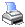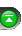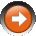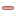﻿ Statistics Definitions
 Operations > Control Center > Account Performance Tab > Statistics DefinitionsThe following are definitions and formulas used for Account Performance statistics:Understanding Average Time in Market
 Average Time in Market This statistic returns a value that gives you an idea of how long you can expect your positions to be open. You can use this by manually closing out a position if you feel it has been in the market for too long.   SUM(exit date – entry date) of all trades / # of tradesUnderstanding Average ETD

Average ETD

This statistic returns a value that is useful in giving you a measure of how effective your exit conditions capture the price movements after your strategy enters a position. It shows you how much you give back from the best price reached before you exit the trade. A small number here is generally desirable since it would imply highly optimized exit conditions that capture most of the price movement you were after.

 Currency Average MFE – Average Trade Percent Average MFE – Average Trade Points Average MFE – Average TradeUnderstanding Average Losing Trade

This statistic returns a value representing the average loss you experience from all of your losing trades. It is useful for getting an idea of how much you could expect to lose on losing trades.

 Currency SUM(loss * quantity * point value) of all losing trades / # of losing trades Percent SUM(profit * quantity / entry price) of all losing trades / # of losing traded lots Points SUM(profit * quantity) of all losing trades / # of losing trades

where loss is defined as (exit price – entry price) for long trades and as (entry price – exit price) for short trades, and point value is defined as the monetary conversion of each point (e.g. 100 for currency pairs).Understanding Average MAE

Average MAE

This statistic returns a value representing the average maximum run-down your strategy experiences. This information helps you gauge how poorly your strategy’s entry conditions predict upcoming price movement directions. A low percentage here is desirable since it would imply that the price movement after you enter a position follows the direction of your intended trade.

 Currency SUM(MAE * quantity * point value) of all trades / # of trades Percent SUM(MAE * quantity / entry price) of all trades / # of traded lots Points SUM(MAE * quantity) of all trades / # of trades

where MAE (max. adverse excursion) is defined as |worst price trade reached – entry price|, quantity is defined as the number of contracts traded, and point value is defined as the monetary conversion of each point (e.g. 100 for currency pairs).Understanding Average MFE

Average MFE

This statistic returns a value representing the average maximum run-up your strategy experiences. This information helps you gauge how well your strategy’s entry conditions predict upcoming price movements. A high percentage here is desirable since it would imply high profitability opportunities.

 Currency SUM(MFE * quantity * point value) of all trades / # of trades Percent SUM(MFE * quantity / entry price) of all trades / # of traded lots Points SUM(MFE * quantity) of all trades / # of trades

where MFE (max. favorable excursion) is defined as (best price trade reached – entry price), quantity is defined as the number of contracts traded, and point value is defined as the monetary conversion of each point (e.g. 100 for currency pairs).Understanding Average Trade

This statistic returns a value representing the average profit you experience from all of your trades. It is useful for getting an idea of how much you could expect to earn on future trades.

 Currency SUM(profit * quantity * point value) of all trades / # of trades Percent SUM(profit * quantity / entry price) of all trades / # of traded lots Points SUM(profit * quantity) of all trades / # of trades

where profit is defined as (exit price – entry price) for long trades and as (entry price – exit price) for short trades, quantity is defined as the number of contracts traded, and point value is defined as the monetary conversion of each point (e.g. 100 for currency pairs).Understanding Average Winning Trade

This statistic returns a value representing the average profit you experience from all of your winning trades. It is useful for getting an idea of how much you could expect to earn on winning trades.

 Currency SUM(profit * quantity * point value) of all winning trades / # of winning trades Percent SUM(profit * quantity / entry price) of all winning trades / # of winning traded lots Points SUM(profit * quantity) of all winning trades / # of winning trades

where profit is defined as (exit price – entry price) for long trades and as (entry price – exit price) for short trades, quantity is defined as the number of contracts traded, and point value is defined as the monetary conversion of each point (e.g. 100 for currency pairs).Understanding Commission
 Commission This statistic returns a value that is the sum of all the commission fees associated with the trades executed by the strategy.   SUM(commission of all traded executions)Understanding Cumulative Profit

Cumulative profit

This statistic returns a value representing a summation of all the profits earned by all your trades. It can be interpreted as a performance measure for your strategy.

 Currency SUM(profit * quantity * point value) of all trades Percent PRODUCT(1 + profit / entry price) of all trades - 1 Points SUM(profit * quantity) of all trades

where profit is defined as (exit price – entry price) for long trades and as (entry price – exit price) for short trades, quantity is defined as the number of contracts traded, and point value is defined as the monetary conversion of each point (e.g. 100 for currency pairs).Understanding Gross Loss
 Gross Loss This statistic returns a monetary value representing a summation of all the money lost across all your trades with your strategy.   SUM(loss * quantity) of all losing trades where loss is defined as (exit price – entry price) for long trades and as (entry price – exit price) for short trades and quantity is defined as the number of contracts traded.Understanding Gross Profit
 Gross Profit This statistic returns a monetary value representing a summation of all the money earned across all your trades with your strategy.   SUM(profit * quantity) of all winning trades where profit is defined as (exit price – entry price) for long trades and as (entry price – exit price) for short trades and quantity is defined as the number of contracts traded.Understanding Max. Drawdown
 Max. Drawdown The maximum drawdown statistic provides you with information regarding the biggest decrease (drawdown) in account size experienced by your strategy. Drawdown is often used as an indicator of risk.   Drawdown = local maximum account size – local minimum account size Max Drawdown = single largest Drawdown   As an example, your account rises from \$25,000 to \$50,000. It then subsequently drops to \$40,000 but rises again to \$60,000. The drawdown in this case would be \$10,000 or -20%. Take note that drawdown does not necessarily have to correspond with a loss in your original account principal.Understanding Profit Factor
 Profit Factor This statistic returns a ratio that can be used as a performance measure for your strategy. It gives you an idea of how much more money your strategy earns then it loses. A higher ratio can be considered characteristic of a high performing strategy. A ratio less than one indicates your strategy loses more money than it earns.   Gross Profit / Gross LossUnderstanding Profit Per Month

Profit Per Month

This statistic returns a value that can be used as a performance measure for your strategy. It gives you an idea of how much profit you can expect to make per month.

 Currency cumulative profit / # of months Percent (1 + cumulative profit)(1 / # of months) - 1 Points cumulative profit / # of months

(See the cumulative profit statistic above for its definition.)Understanding Ratio Avg Win / Avg Loss
 Ratio Avg Win / Avg Loss This statistic returns a ratio that can be used as a performance measure for your strategy. A value greater than 1 signifies you win more than you lose. A value less than 1 signifies you lose more than you win.   Average Winning Trade / Average Losing TradeUnderstanding Sharpe Ratio

Sharpe Ratio

This statistic returns a ratio that measures the risk premium per unit of risk of your strategy. It can help you make decisions based on the excess risk of your strategies. You may have a high-return strategy, but the high returns may come at a cost of excess risk. The Sharpe ratio will help you determine if it is an appropriate increase in risk for the higher return or not. Generally, a ratio of 1 or greater is good, 2 or greater is very good, and 3 and up is great.

(Profit per Month – risk free Rate of Return) / standard deviation of monthly profits

Note:

 • NinjaTrader presets "risk free Rate of Return" to a value of zero
 • In the event that there is only 1 month of trade history or less, there is insufficient data to calculate the monthly standard deviation of profits in which event, the Sharpe Ratio is set to a value of 1

(See the Profit Per Month statistic above for its definition.)